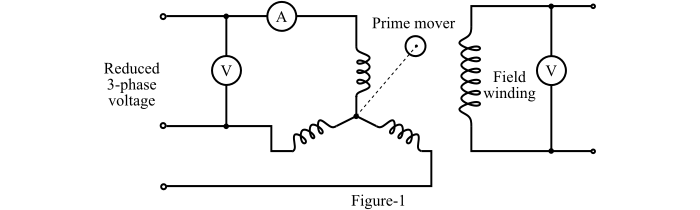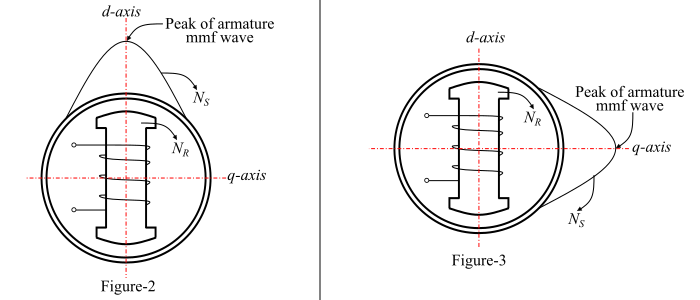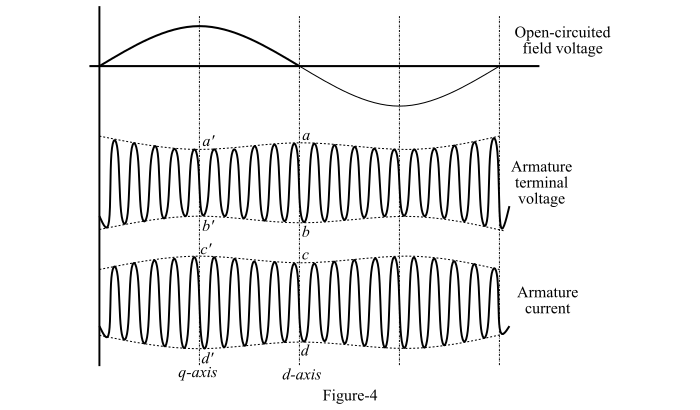# Slip Test on Synchronous Machine

The slip test is a simple no-load test, which is used to determine the direct-axis and quadrature-axis synchronous reactances of a salient-pole synchronous machine. In this test, a small voltage at rated frequency is applied to the 3-phase stator winding of the synchronous machine. The field winding is unexcited and left open circuited.

## Circuit Arrangement for Slip Test

The circuit arrangement for slip test is shown in Figure-1.The rotor is driven by an auxiliary motor at a speed slightly less than or more than the synchronous speed. The direction of rotation should be same as that of the rotating magnetic field produced by the stator.

A small voltage reading indicated by the voltmeter connected across the open circuited field winding terminals shows that the direction of the rotation of the rotor is proper.

The rotor is running at a speed ($𝑁_{𝑅}$) close to the synchronous speed ($𝑁_{𝑆}$) and there will be a small slip between the rotating magnetic field produced by the armature and the actual salient-field poles. Thus, the relative speed between the armature MMF and the salient field poles is equal to the slip speed ($𝑁_{𝑆}$ − $𝑁_{𝑅}$).

Since the armature MMF moves slowly past the actual field poles, hence there will be an instant when the peak of the armature MMF wave is in line with the axis of the actual salient field poles as shown in Figure-2.The axis of the field poles is direct axis or d-axis. In this position, the reluctance offered by the small air gap is minimum and this results in a minimum magnetising current 𝐼𝑚𝑖𝑛 indicated by the line ammeter A.

In this position, the armature flux linkage with the field winding is maximum and the rate of change of this flux linkage is zero. Hence, the voltage induced in the field winding is zero. Therefore, the d-axis can be located on the oscillogram as shown in Figure-4.From this figure, the direct axis synchronous reluctance is given by,

$$\mathrm{𝑋_{𝑑} =\frac{𝑎𝑏}{𝑐𝑑}}$$

After one-quarter of slip cycle the peak armature wave is in line with the quadrature axis. In this position, the reluctance offered by the larger air gap is maximum as shown in Figure-3.

Due to the high reluctance, a large magnetising current is required to produce the same air gap flux. This maximum current (𝐼𝑚𝑎𝑥) is also measured from the line ammeter A. In this position, the armature flux linkage with the field winding is also zero and the rate of change of this flux linkage is maximum. As a result, the induced voltage across the field winding is maximum. Therefore, the q-axis can also be located on the oscillogram of Figure-4. From this figure, the quadrature axis synchronous reactance is given by,

$$\mathrm{𝑋_{𝑞} =\frac{{𝑎^{′}}{𝑏^{′}}}{𝑐^{′}𝑑^{′}}}$$